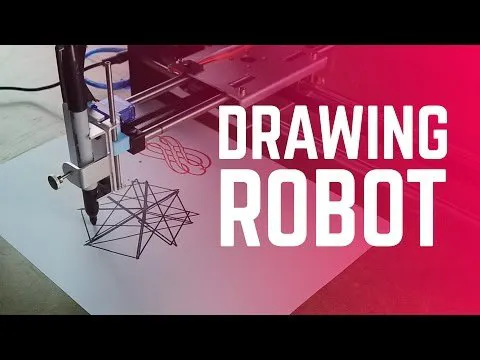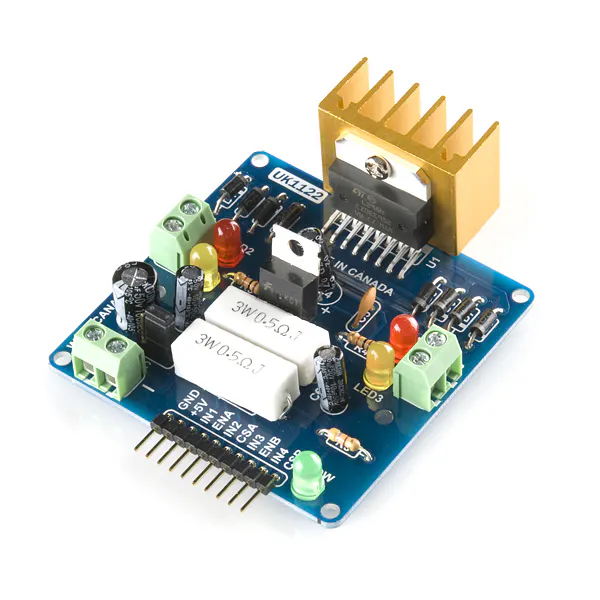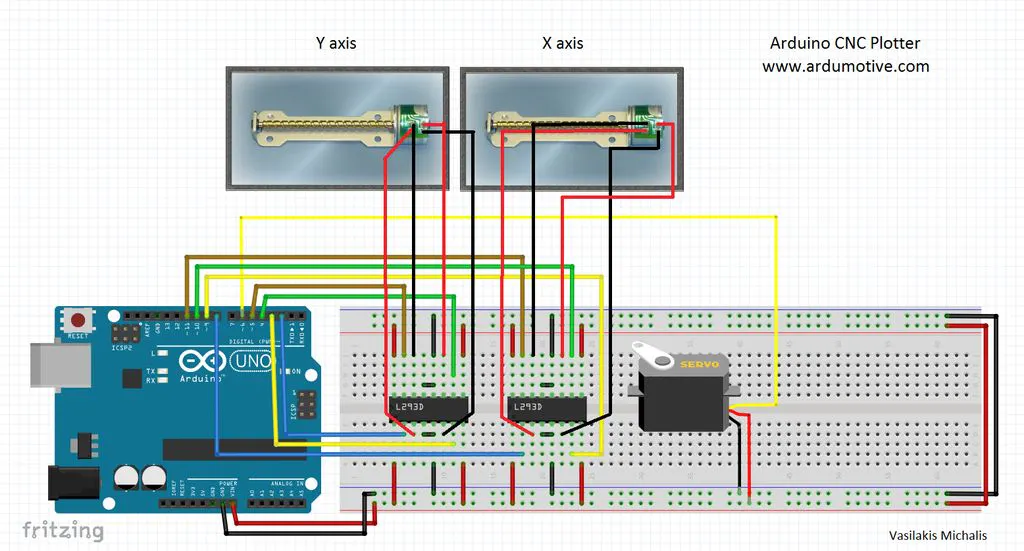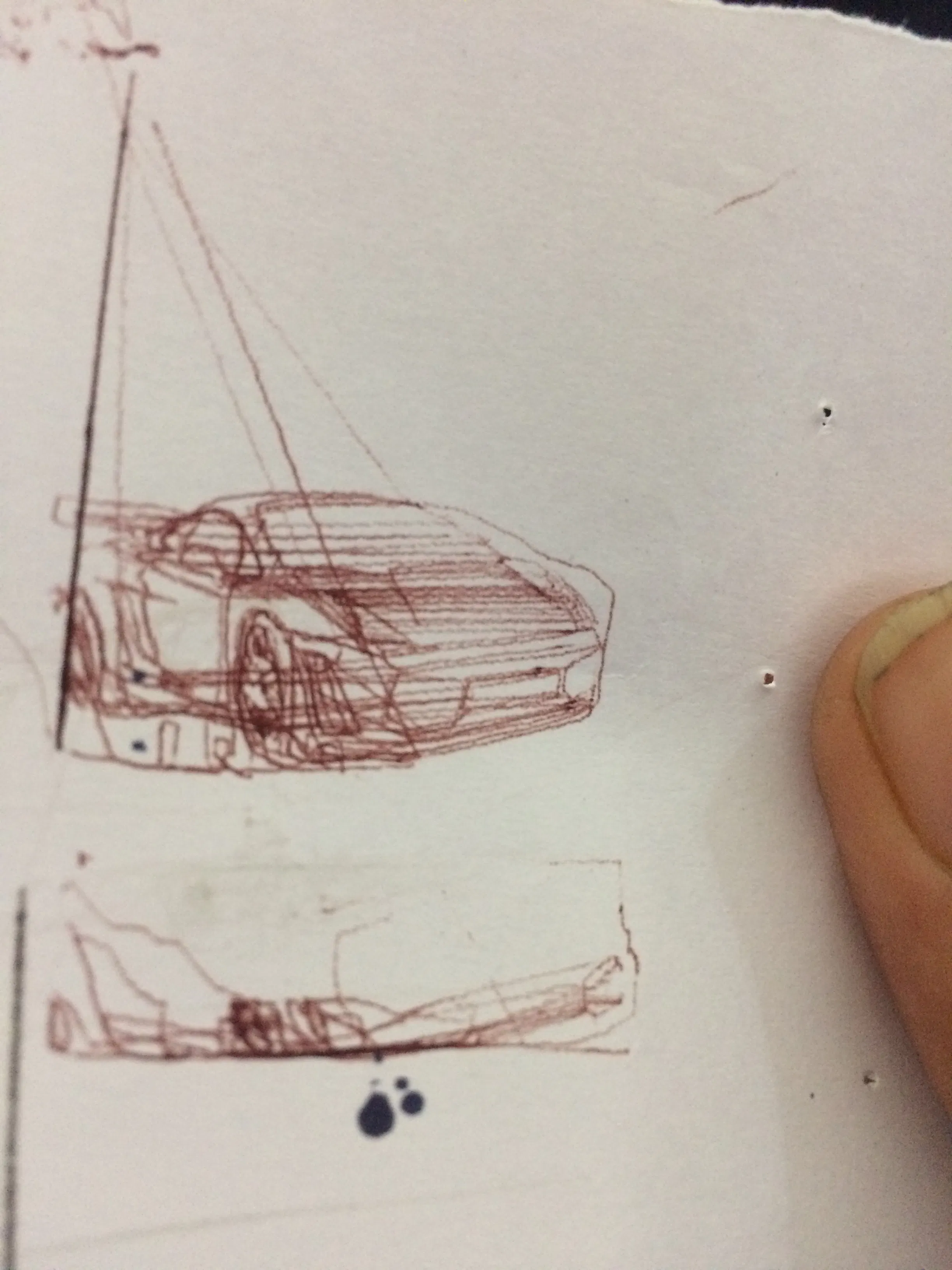Published

# Sketch It (CNC Plotter)

It can sketch accurately, you just have to upload the gcode of the image. Try it it's just awesome.

IntermediateFull instructions provided4 hours130,976## Things used in this project

### Hardware components

 Scrap DVD Writers
×2
 Micro Servo Motor
×1SparkFun Dual H-Bridge motor drivers L298
×2Arduino UNO & Genuino UNO
×1

### Software apps and online servicesArduino IDE
 processing
 Inkscape Vector Graphics Editor

## Schematics

plug in wires in the arduino only after removing power### my first print an Avantador## Code

### arduino code

Arduino
```/*
Mini CNC Plotter firmware, based in TinyCNC https://github.com/MakerBlock/TinyCNC-Sketches
Send GCODE to this Sketch using gctrl.pde https://github.com/damellis/gctrl
Convert SVG to GCODE with MakerBot Unicorn plugin for Inkscape available here https://github.com/martymcguire/inkscape-unicorn

*/

#include <Servo.h>
#include <Stepper.h>

#define LINE_BUFFER_LENGTH 512

// Servo position for Up and Down
const int penZUp = 80;
const int penZDown = 40;

// Servo on PWM pin 6
const int penServoPin = 6;

// Should be right for DVD steppers, but is not too important here
const int stepsPerRevolution = 20;

// create servo object to control a servo
Servo penServo;

// Initialize steppers for X- and Y-axis using this Arduino pins for the L293D H-bridge
Stepper myStepperY(stepsPerRevolution, 2,3,4,5);
Stepper myStepperX(stepsPerRevolution, 8,9,10,11);

/* Structures, global variables    */
struct point {
float x;
float y;
float z;
};

struct point actuatorPos;

//  Drawing settings, should be OK
float StepInc = 1;
int StepDelay = 0;
int LineDelay = 50;
int penDelay = 50;

// Motor steps to go 1 millimeter.
// Use test sketch to go 100 steps. Measure the length of line.
// Calculate steps per mm. Enter here.
float StepsPerMillimeterX = 6.0;
float StepsPerMillimeterY = 6.0;

// Drawing robot limits, in mm
// OK to start with. Could go up to 50 mm if calibrated well.
float Xmin = 0;
float Xmax = 40;
float Ymin = 0;
float Ymax = 40;
float Zmin = 0;
float Zmax = 1;

float Xpos = Xmin;
float Ypos = Ymin;
float Zpos = Zmax;

// Set to true to get debug output.
boolean verbose = false;

//  Needs to interpret
//  G1 for moving
//  G4 P300 (wait 150ms)
//  M300 S30 (pen down)
//  M300 S50 (pen up)
//  Discard anything with a (

/**********************
* void setup() - Initialisations
***********************/
void setup() {
//  Setup
Serial.begin( 9600 );

penServo.attach(penServoPin);
penServo.write(penZUp);
delay(200);

// Decrease if necessary
myStepperX.setSpeed(250);
myStepperY.setSpeed(250);

//  Set & move to initial default position
// TBD

Serial.println("Mini CNC Plotter alive and kicking!");
Serial.print("X range is from ");
Serial.print(Xmin);
Serial.print(" to ");
Serial.print(Xmax);
Serial.println(" mm.");
Serial.print("Y range is from ");
Serial.print(Ymin);
Serial.print(" to ");
Serial.print(Ymax);
Serial.println(" mm.");
}

/**********************
* void loop() - Main loop
***********************/
void loop()
{
delay(200);
char line[ LINE_BUFFER_LENGTH ];
char c;
int lineIndex;
bool lineIsComment, lineSemiColon;

lineIndex = 0;
lineSemiColon = false;
lineIsComment = false;

while (1) {

// Serial reception - Mostly from Grbl, added semicolon support
while ( Serial.available()>0 ) {
if (( c == '\n') || (c == '\r') ) {             // End of line reached
if ( lineIndex > 0 ) {                        // Line is complete. Then execute!
line[ lineIndex ] = '\0';                   // Terminate string
if (verbose) {
Serial.println( line );
}
processIncomingLine( line, lineIndex );
lineIndex = 0;
}
else {
// Empty or comment line. Skip block.
}
lineIsComment = false;
lineSemiColon = false;
Serial.println("ok");
}
else {
if ( (lineIsComment) || (lineSemiColon) ) {   // Throw away all comment characters
if ( c == ')' )  lineIsComment = false;     // End of comment. Resume line.
}
else {
if ( c <= ' ' ) {                           // Throw away whitepace and control characters
}
else if ( c == '/' ) {                    // Block delete not supported. Ignore character.
}
else if ( c == '(' ) {                    // Enable comments flag and ignore all characters until ')' or EOL.
lineIsComment = true;
}
else if ( c == ';' ) {
lineSemiColon = true;
}
else if ( lineIndex >= LINE_BUFFER_LENGTH-1 ) {
Serial.println( "ERROR - lineBuffer overflow" );
lineIsComment = false;
lineSemiColon = false;
}
else if ( c >= 'a' && c <= 'z' ) {        // Upcase lowercase
line[ lineIndex++ ] = c-'a'+'A';
}
else {
line[ lineIndex++ ] = c;
}
}
}
}
}
}

void processIncomingLine( char* line, int charNB ) {
int currentIndex = 0;
char buffer[ 64 ];                                 // Hope that 64 is enough for 1 parameter
struct point newPos;

newPos.x = 0.0;
newPos.y = 0.0;

//  Needs to interpret
//  G1 for moving
//  G4 P300 (wait 150ms)
//  G1 X60 Y30
//  G1 X30 Y50
//  M300 S30 (pen down)
//  M300 S50 (pen up)
//  Discard anything with a (

while( currentIndex < charNB ) {
switch ( line[ currentIndex++ ] ) {              // Select command, if any
case 'U':
penUp();
break;
case 'D':
penDown();
break;
case 'G':
buffer = line[ currentIndex++ ];          // /!\ Dirty - Only works with 2 digit commands
//      buffer = line[ currentIndex++ ];
//      buffer = '\0';
buffer = '\0';

switch ( atoi( buffer ) ){                   // Select G command
case 0:                                   // G00 & G01 - Movement or fast movement. Same here
case 1:
// /!\ Dirty - Suppose that X is before Y
char* indexX = strchr( line+currentIndex, 'X' );  // Get X/Y position in the string (if any)
char* indexY = strchr( line+currentIndex, 'Y' );
if ( indexY <= 0 ) {
newPos.x = atof( indexX + 1);
newPos.y = actuatorPos.y;
}
else if ( indexX <= 0 ) {
newPos.y = atof( indexY + 1);
newPos.x = actuatorPos.x;
}
else {
newPos.y = atof( indexY + 1);
indexY = '\0';
newPos.x = atof( indexX + 1);
}
drawLine(newPos.x, newPos.y );
//        Serial.println("ok");
actuatorPos.x = newPos.x;
actuatorPos.y = newPos.y;
break;
}
break;
case 'M':
buffer = line[ currentIndex++ ];        // /!\ Dirty - Only works with 3 digit commands
buffer = line[ currentIndex++ ];
buffer = line[ currentIndex++ ];
buffer = '\0';
switch ( atoi( buffer ) ){
case 300:
{
char* indexS = strchr( line+currentIndex, 'S' );
float Spos = atof( indexS + 1);
//          Serial.println("ok");
if (Spos == 30) {
penDown();
}
if (Spos == 50) {
penUp();
}
break;
}
case 114:                                // M114 - Repport position
Serial.print( "Absolute position : X = " );
Serial.print( actuatorPos.x );
Serial.print( "  -  Y = " );
Serial.println( actuatorPos.y );
break;
default:
Serial.print( "Command not recognized : M");
Serial.println( buffer );
}
}
}

}

/*********************************
* Draw a line from (x0;y0) to (x1;y1).
* Bresenham algo from https://www.marginallyclever.com/blog/2013/08/how-to-build-an-2-axis-arduino-cnc-gcode-interpreter/
* int (x1;y1) : Starting coordinates
* int (x2;y2) : Ending coordinates
**********************************/
void drawLine(float x1, float y1) {

if (verbose)
{
Serial.print("fx1, fy1: ");
Serial.print(x1);
Serial.print(",");
Serial.print(y1);
Serial.println("");
}

//  Bring instructions within limits
if (x1 >= Xmax) {
x1 = Xmax;
}
if (x1 <= Xmin) {
x1 = Xmin;
}
if (y1 >= Ymax) {
y1 = Ymax;
}
if (y1 <= Ymin) {
y1 = Ymin;
}

if (verbose)
{
Serial.print("Xpos, Ypos: ");
Serial.print(Xpos);
Serial.print(",");
Serial.print(Ypos);
Serial.println("");
}

if (verbose)
{
Serial.print("x1, y1: ");
Serial.print(x1);
Serial.print(",");
Serial.print(y1);
Serial.println("");
}

//  Convert coordinates to steps
x1 = (int)(x1*StepsPerMillimeterX);
y1 = (int)(y1*StepsPerMillimeterY);
float x0 = Xpos;
float y0 = Ypos;

//  Let's find out the change for the coordinates
long dx = abs(x1-x0);
long dy = abs(y1-y0);
int sx = x0<x1 ? StepInc : -StepInc;
int sy = y0<y1 ? StepInc : -StepInc;

long i;
long over = 0;

if (dx > dy) {
for (i=0; i<dx; ++i) {
myStepperX.step(sx);
over+=dy;
if (over>=dx) {
over-=dx;
myStepperY.step(sy);
}
delay(StepDelay);
}
}
else {
for (i=0; i<dy; ++i) {
myStepperY.step(sy);
over+=dx;
if (over>=dy) {
over-=dy;
myStepperX.step(sx);
}
delay(StepDelay);
}
}

if (verbose)
{
Serial.print("dx, dy:");
Serial.print(dx);
Serial.print(",");
Serial.print(dy);
Serial.println("");
}

if (verbose)
{
Serial.print("Going to (");
Serial.print(x0);
Serial.print(",");
Serial.print(y0);
Serial.println(")");
}

//  Delay before any next lines are submitted
delay(LineDelay);
//  Update the positions
Xpos = x1;
Ypos = y1;
}

//  Raises pen
void penUp() {
penServo.write(penZUp);
delay(LineDelay);
Zpos=Zmax;
if (verbose) {
Serial.println("Pen up!");
}
}
//  Lowers pen
void penDown() {
penServo.write(penZDown);
delay(LineDelay);
Zpos=Zmin;
if (verbose) {
Serial.println("Pen down.");
}
}
```

### gctrl code(processing)

Arduino
To send gcode to the plotter (Processing)
```import java.awt.event.KeyEvent;
import javax.swing.JOptionPane;
import processing.serial.*;

Serial port = null;

// select and modify the appropriate line for your operating system
// leave as null to use interactive port (press 'p' in the program)
String portname = null;
//String portname = Serial.list(); // Mac OS X
//String portname = "/dev/ttyUSB0"; // Linux
//String portname = "COM6"; // Windows

boolean streaming = false;
float speed = 0.001;
String[] gcode;
int i = 0;

void openSerialPort()
{
if (portname == null) return;
if (port != null) port.stop();

port = new Serial(this, portname, 9600);

port.bufferUntil('\n');
}

void selectSerialPort()
{
String result = (String) JOptionPane.showInputDialog(frame,
"Select the serial port that corresponds to your Arduino board.",
"Select serial port",
JOptionPane.QUESTION_MESSAGE,
null,
Serial.list(),
0);

if (result != null) {
portname = result;
openSerialPort();
}
}

void setup()
{
size(500, 250);
openSerialPort();
}

void draw()
{
background(0);
fill(255);
int y = 24, dy = 12;
text("INSTRUCTIONS", 12, y); y += dy;
text("p: select serial port", 12, y); y += dy;
text("1: set speed to 0.001 inches (1 mil) per jog", 12, y); y += dy;
text("2: set speed to 0.010 inches (10 mil) per jog", 12, y); y += dy;
text("3: set speed to 0.100 inches (100 mil) per jog", 12, y); y += dy;
text("arrow keys: jog in x-y plane", 12, y); y += dy;
text("page up & page down: jog in z axis", 12, y); y += dy;
text("\$: display grbl settings", 12, y); y+= dy;
text("h: go home", 12, y); y += dy;
text("0: zero machine (set home to the current location)", 12, y); y += dy;
text("g: stream a g-code file", 12, y); y += dy;
text("x: stop streaming g-code (this is NOT immediate)", 12, y); y += dy;
y = height - dy;
text("current jog speed: " + speed + " inches per step", 12, y); y -= dy;
text("current serial port: " + portname, 12, y); y -= dy;
}

void keyPressed()
{
if (key == '1') speed = 0.001;
if (key == '2') speed = 0.01;
if (key == '3') speed = 0.1;

if (!streaming) {
if (keyCode == LEFT) port.write("G91\nG20\nG00 X-" + speed + " Y0.000 Z0.000\n");
if (keyCode == RIGHT) port.write("G91\nG20\nG00 X" + speed + " Y0.000 Z0.000\n");
if (keyCode == UP) port.write("G91\nG20\nG00 X0.000 Y" + speed + " Z0.000\n");
if (keyCode == DOWN) port.write("G91\nG20\nG00 X0.000 Y-" + speed + " Z0.000\n");
if (keyCode == KeyEvent.VK_PAGE_UP) port.write("G91\nG20\nG00 X0.000 Y0.000 Z" + speed + "\n");
if (keyCode == KeyEvent.VK_PAGE_DOWN) port.write("G91\nG20\nG00 X0.000 Y0.000 Z-" + speed + "\n");
if (key == 'h') port.write("G90\nG20\nG00 X0.000 Y0.000 Z0.000\n");
if (key == 'v') port.write("\$0=75\n\$1=74\n\$2=75\n");
//if (key == 'v') port.write("\$0=100\n\$1=74\n\$2=75\n");
if (key == 's') port.write("\$3=10\n");
if (key == 'e') port.write("\$16=1\n");
if (key == 'd') port.write("\$16=0\n");
if (key == '0') openSerialPort();
if (key == 'p') selectSerialPort();
if (key == '\$') port.write("\$\$\n");
}

if (!streaming && key == 'g') {
gcode = null; i = 0;
File file = null;
selectInput("Select a file to process:", "fileSelected", file);
}

if (key == 'x') streaming = false;
}

void fileSelected(File selection) {
if (selection == null) {
println("Window was closed or the user hit cancel.");
} else {
println("User selected " + selection.getAbsolutePath());
if (gcode == null) return;
streaming = true;
stream();
}
}

void stream()
{
if (!streaming) return;

while (true) {
if (i == gcode.length) {
streaming = false;
return;
}

if (gcode[i].trim().length() == 0) i++;
else break;
}

println(gcode[i]);
port.write(gcode[i] + '\n');
i++;
}

void serialEvent(Serial p)
{
println(s.trim());

if (s.trim().startsWith("ok")) stream();
if (s.trim().startsWith("error")) stream(); // XXX: really?
}
```

## Credits

### Yogeshmodi

2 projects • 50 followers